# Asymptotic line

A curveon a regular surfacesuch that the normal curvature alongis zero. An asymptotic line is given by the differential equation: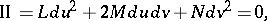where II is the second fundamental form of the surface.

The osculating plane of an asymptotic line, if it exists, coincides with the tangent plane to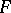(at the points of), and the square of the torsion of an asymptotic line is equal to the modulus of the Gaussian curvatureof the surface(the Beltrami–Enneper theorem). A straight line(e.g. a generating line of a ruled surface) is always an asymptotic line. Ifis a parabolic curve (e.g. a circle on a standard torus separating the domains of Gaussian curvatures of different signs), it is an asymptotic line.

A unique asymptotic line, which coincides with the rectilinear generator, passes through each point of a parabolic domain (where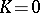, but II). Through each point of a hyperbolic domain (where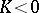) there pass exactly two asymptotic lines, forming the so-called asymptotic net, which plays an important role in the study of the spatial form of a surface of negative curvature (cf. Negative curvature, surface of). For instance, on a complete surface this net is homeomorphic to the Cartesian net on the plane if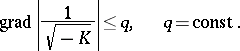Asymptotic nets on surfaces of constant negative curvature are Chebyshev nets (cf. Chebyshev net), and the surface area of a quadrangle formed by asymptotic lines is proportional to the excess of the sum of its interior anglesover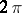: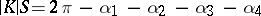(Hazzidakis' formula).

Under a projective transformationof the space, the asymptotic lines of a surfacebecome the asymptotic lines of the transformed surface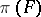.

Asymptotic lines on surfaces in a three-dimensional Riemannian space are defined in a similar manner. Various generalizations of the concept of an asymptotic line on manifolds imbedded in a multi-dimensional space are known; the most frequently-used one involves the concept of the second fundamental form, which is associated with a given normal vector.